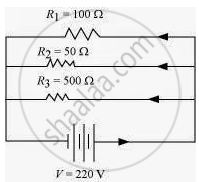# An electric lamp of 100 Ω, a toaster of resistance 50 Ω, and a water filter of resistance 500 Ω are connected in parallel to a 220 Vsource. - Science and Technology 1

An electric lamp of 100 Ω, a toaster of resistance 50 Ω, and a water filter of resistance 500 Ω are connected in parallel to a 220 Vsource. What is the resistance of an electric iron connected to the same source that takes as much current as all three appliances, and what is the current through it?

#### Solution

Resistance of electric lamp, R1 = 100 Ω
Resistance of toaster, R2 = 50 Ω
Resistance of water filter, R3 = 500 Ω
Potential difference of the source, V = 220 V
These are connected in parallel, as shown in the following figureLet R be the equivalent resistance of the circuit.

The net resistance in parallel is given by

1/R=1/R_1+1/R_2+1/R_3

Here, R1 = 100 Ω, R2 = 50 Ω and R3 = 500 Ω

1/R=1/100+1/50+1/500

=(5+10+1)/500=16/500

R=500/16=31.25 Ω

Now, using Ohm’s law V = IR, we have

I =V/R

=(220 V)/(31.25 Ω)

= 7.04 A

Hence, the resistance of electric iron is 31.25 Ω and current through it is 7.04 A.

Concept: System of Resistors - Resistances in Parallel
Is there an error in this question or solution?

#### APPEARS IN

NCERT Class 10 Science
Chapter 12 Electricity
Q 2 | Page 216